﻿ Changing octave bandwidth in formulae### How To "Strutt" Series

Changing octave bandwidth in formulae    1/1, 1/3, 1/2, 1/4

It is possible to directly edit Strutt formulae that have been inserted into a worksheet. This example will focus on changing the bandwidth parameter, i.e. the parameter in the formula that dictates whether the output relates to 1/1 octave bands or 1/3 octave bands.

To illustrate, the ISOFreqs() Strutt formula is inserted into a Strutt sheet via Fundamentals->ISO Bandwidth (Figure 1). In this example, the selected row is on a 1/1 octave band Strutt sheet.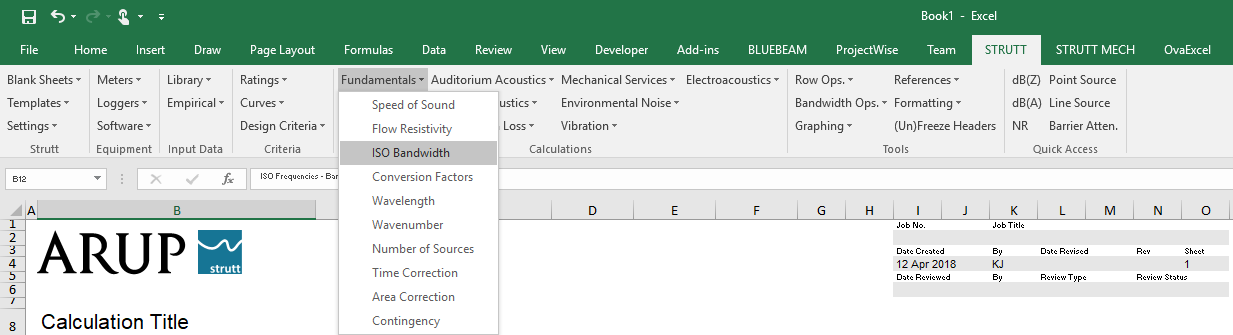Figure 1

The ISO Bandwidth form inserts the bandwidth, centre frequency (exact or preferred) or cutoff frequencies (lower or upper) for each standard ISO frequency band into the current row of the worksheet.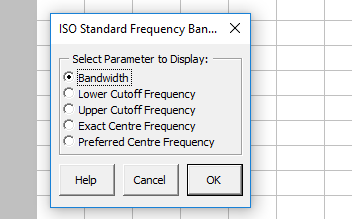Figure 2

Selecting the first radio button on the ISO Bandwidth user form (Figure 2) inserts the bandwidths for the corresponding centre frequencies into the selected row (Figure 3).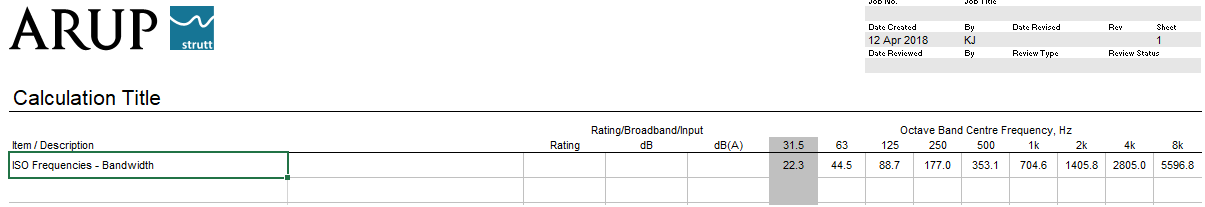Figure 3

Since this is a 1/1 octave band sheet, the form inputs 1/1 octave bandwidths.

If the user would like to view 1/3 octave bandwidths, they could open a 1/3 octave band sheet, open the user form and insert the bandwidths on a row in the 1/3 octave band sheet.

Alternatively, the formula in each cell can simply be updated by changing the value of the last parameter in the excel formula. Since the values in Figure 3 are 1/1 octave bandwidths, the last parameter in the formula is 1 (Figure 4).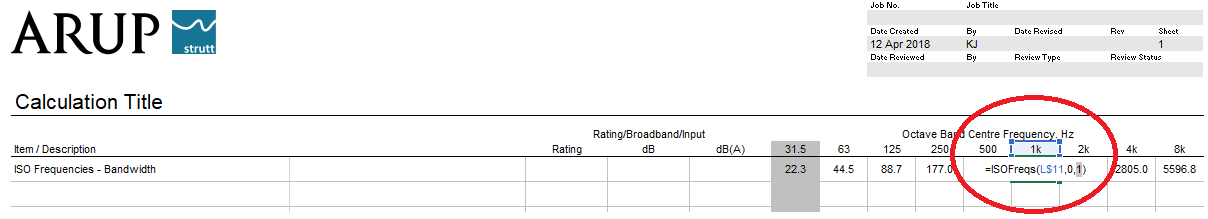Figure 4

By updating this last parameter in the 1 kHz cell to 3, the formula returns the 1/3 octave bandwidth for 1 kHz (Figure 5).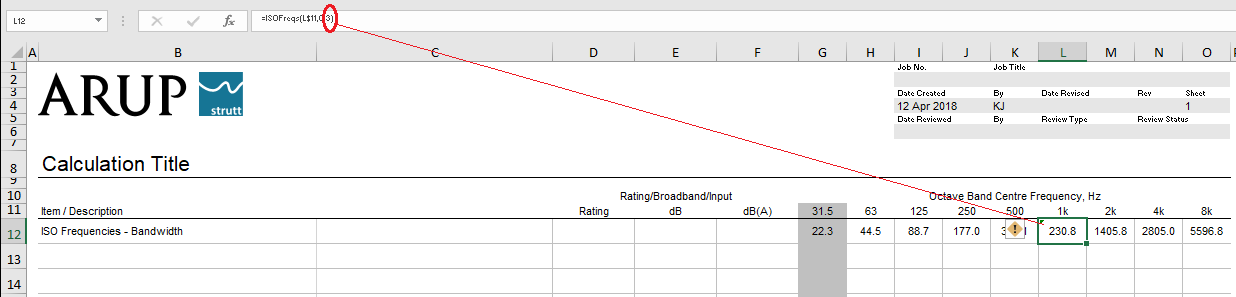Figure 5

In addition, 1/2 octave bandwidths can be returned by changing the last parameter to 2 and 1/4 octave bandwidths can be inserted by changing the last parameter to 4.

This method works for any formula that accepts the bandwidth as a parameter.